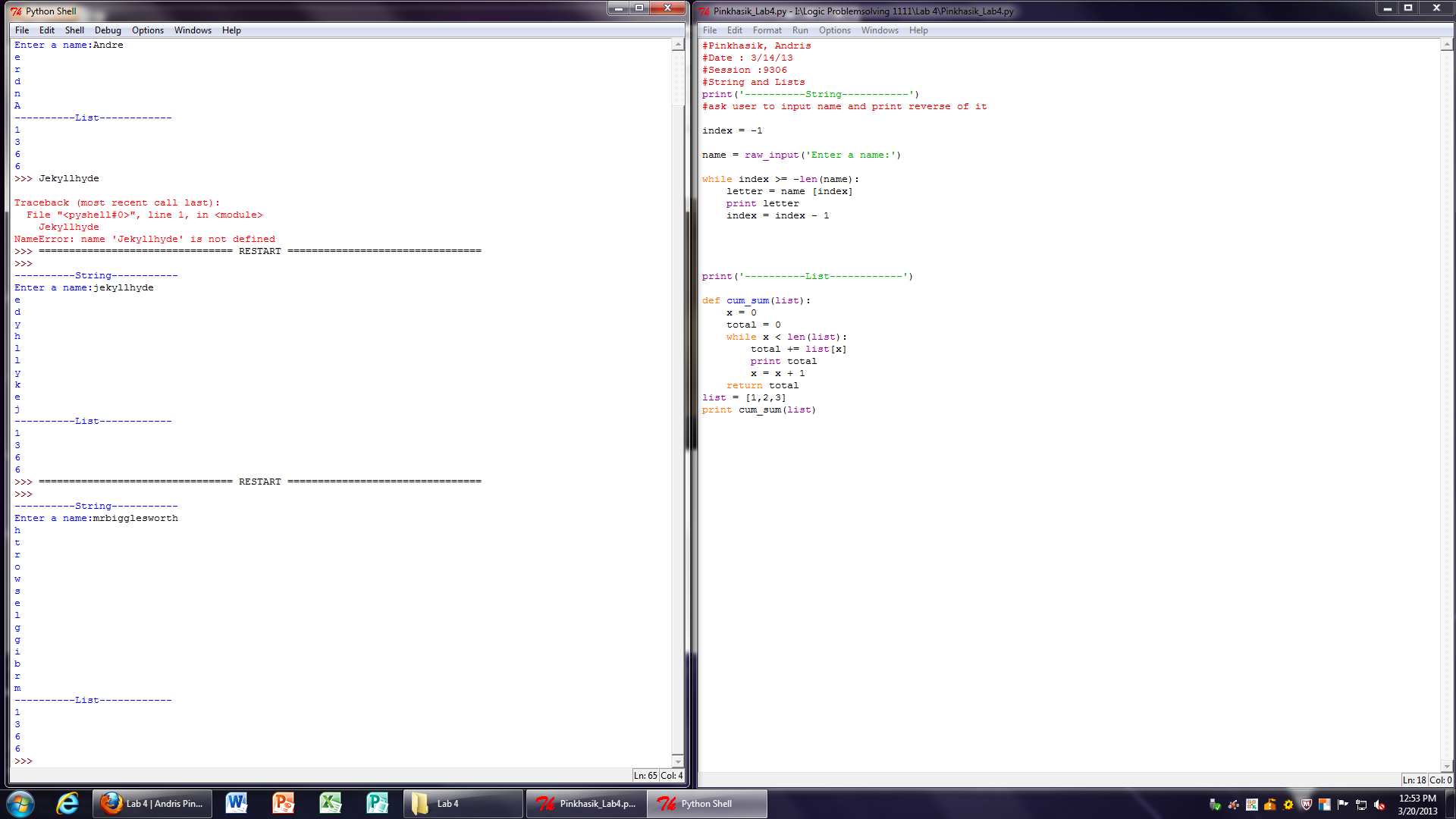# Lab 4

Lab Description:

For chapter 8, exercise 1: I assigned the index to -1. Then I assigned raw_input for name input. The program would take the input name and print it in reverse. While the index is greater or equal to the length of the name, it will print the letter in reverse until it reaches the final letter.

For chapter 10, exercise 3: I created a function called cum_sum which would print the cumulative sum of the list input. While x is less than the length of the list, the program will print the cumulative sum of [1,2,3] which is [1,3,6].

Source Code:

print(‘———-String———–‘)

index = -1

name = raw_input(‘Enter a name:’)

while index >= -len(name):
letter = name [index]
print letter
index = index – 1

print(‘———-List————‘)

def cum_sum(list):
x = 0
total = 0
while x < len(list):
total += list[x]
print total
x = x + 1